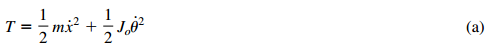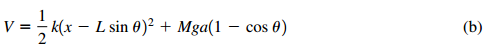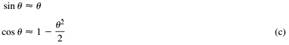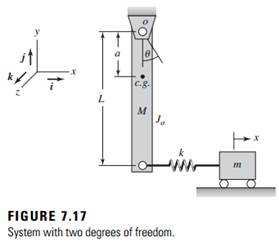### Create an Account

Already have account?

### Forgot Your Password ?

Home / Questions / Repeat Example 715 when ωr 2 0 and Jr 1 0 EXAMPLE 715 Natural frequencies and mode shap...

# Repeat Example 715 when ωr 2 0 and Jr 1 0 EXAMPLE 715 Natural frequencies and mode shapes of a pendulum attached to a translating mass Consider the system shown in Figure 717 We shall derive

Repeat Example 7.15 when ωr = 2.0 and Jr = 1.0.

EXAMPLE 7.15

Natural frequencies and mode shapes of a pendulum attached to a translating mass Consider the system shown in Figure 7.17. We shall derive the governing equations of motion for small |θ| and then use these equations to determine the natural frequencies and mode shapes associated with this system. The equations of motion are obtained by using Lagrange’s equations. We will also illustrate how the expressions for the kinetic energy and the potential energy are appropriately truncated to obtain the linear equations of motion. Considering the translation of the point mass m, and the rotation of the rigid bar M, the system kinetic energy isand the system potential energy iswhere the datum for computing the potential energy due to gravity loading has been chosen at the center of mass of the pendulum. For “small” oscillations about θ = 0, Taylor-series expansions lead toMay 16 2020 View more View Less

#### Answer (Solved)Subscribe To Get Solution